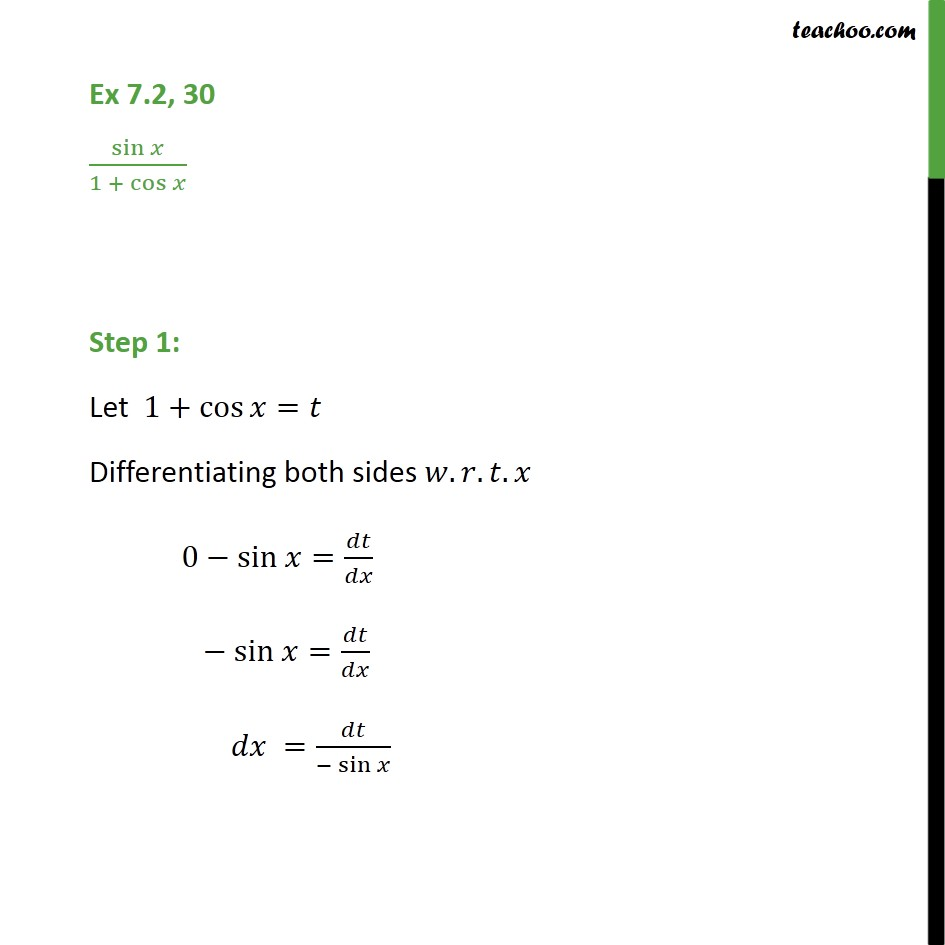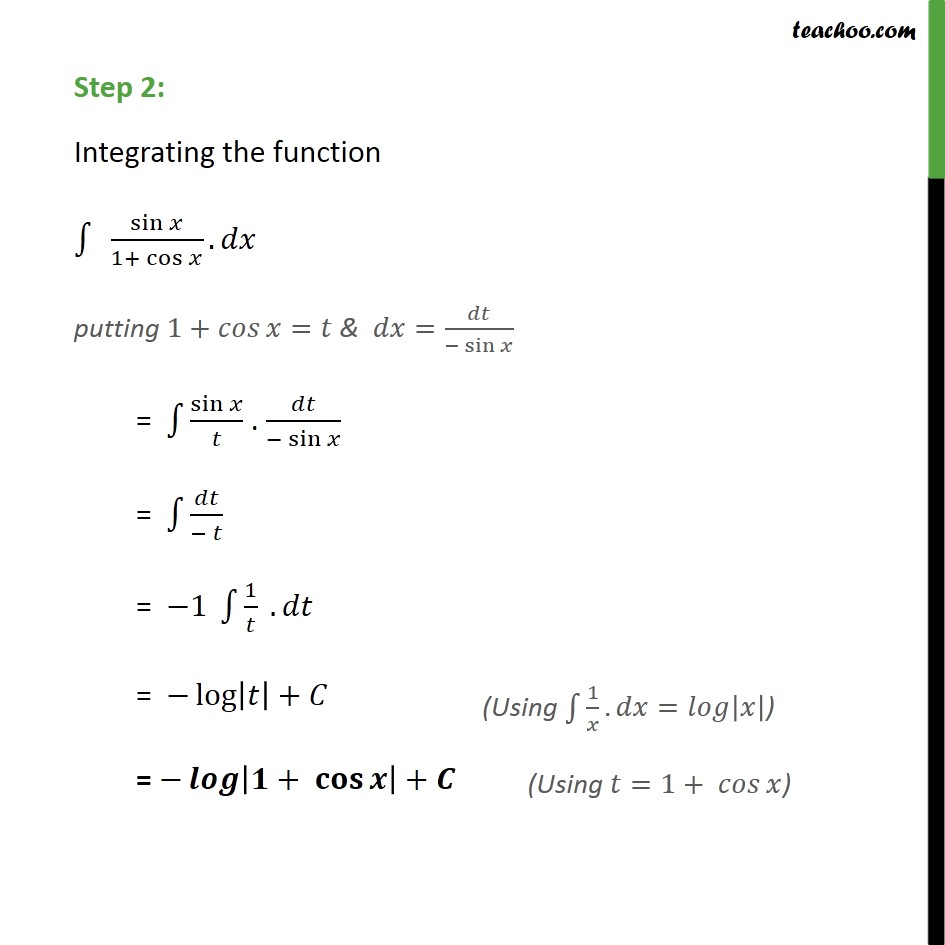1. Chapter 7 Class 12 Integrals
2. Serial order wise
3. Ex 7.2

Transcript

Ex 7.2, 30 sin 1 + cos Step 1: Let 1+ cos = Differentiating both sides . . . 0 sin = sin = = sin Step 2: Integrating the function sin 1+ cos . putting 1+ = & = sin = sin . sin = = 1 1 . = log + = + +

Ex 7.2

Chapter 7 Class 12 Integrals
Serial order wise